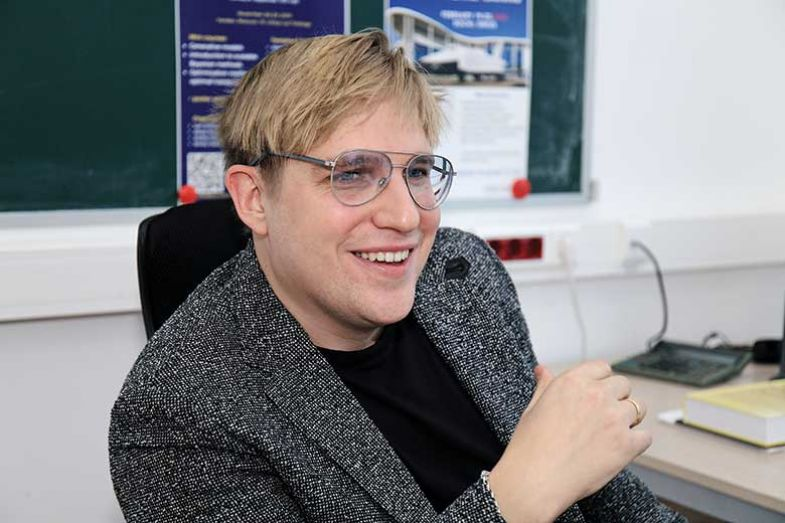HSE UniversityPowering the data revolution with new algorithms and mathematics

# Powering the data revolution with new algorithms and mathematics

### Researchers at HSE University are combining mathematics and computer science to create new algorithms that help mitigate uncertainty in the digital world

We live in the information age, where data are gathered at an unprecedented scale and rate. However, such vast amounts of information are only useful if they can be effectively interpreted, creating new knowledge to solve the world’s problems.

Few people understand that problem better than Alexey Naumov (pictured, below), head of the International Laboratory of Stochastic Algorithms and High-Dimensional Inference, at HSE University in Russia. “In today’s world, we can see information everywhere, accumulated in all possible ways: images, sensors, speech data, large internet networks.

“In most cases, the data are of a stochastic nature. This can be caused by model assumptions, measurement errors, and so on. Our lab aims to develop mathematical methods and algorithms to analyse complex structured data like these,” he says.

Professor Naumov admits that the process of utilising such data to enable scientific understanding is often difficult. “Statistical science provides a principled framework for this process,” he says. “However, as the size and complexity of modern datasets continues to increase, and the understanding sought from the data also expands, fresh challenges emerge to implement formal statistical approaches. Many classical statistical methods do not work for such complex data. So, we need to develop new algorithms and new mathematics to do it.”Source:
HSE University

Much of the laboratory’s work is what Professor Naumov calls the “maths of machine learning”, with potential applications in everything from financial markets to the development of self-driving cars. “The key assumption as the basis of modern approaches to data analysis is that even very complex data have a certain structure,” he explains. “Knowledge of these structures helps [us] to construct efficient algorithms and understand their statistical properties. To extract structural information from the data and use it effectively, we apply modern methods from pure and applied mathematics, such as statistics, probability theory and optimisation theory.”

The laboratory’s recent research includes a project on computational optimal transport, an area of increasing importance in machine learning. “Informally, it is about very quickly calculating transportation distances between probability distributions,” says Professor Naumov. “Image retrieval is a good example. If you Google ‘red car in a yellow field’, you get a Ferrari in a field of wheat and similar pictures.

“How to find similar pictures? One way is to map pictures to colour histograms – ie, probability distribution of colours in an image – calculate the distance between histograms and display the close ones.

“You can think of the probability distribution as a pile of ground. And you would like to transfer one pile into another. The total effort or cost will give you a distance. Computationally, such problems are hard. To do it quickly, you need to develop fast algorithms.”

The laboratory’s results, an efficient computational algorithm for calculating such distances with theoretical guarantees, were presented at the 2018 International Conference on Machine Learning.

Its other projects include reducing uncertainty in machine-learning algorithms, effective dimension reduction for huge datasets and reconstruction geometry of the data.

These results are published in the leading journals on probability, statistics, machine learning and finance.

Find out more about HSE University

Brought to you by